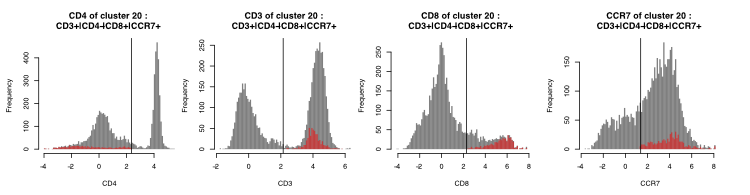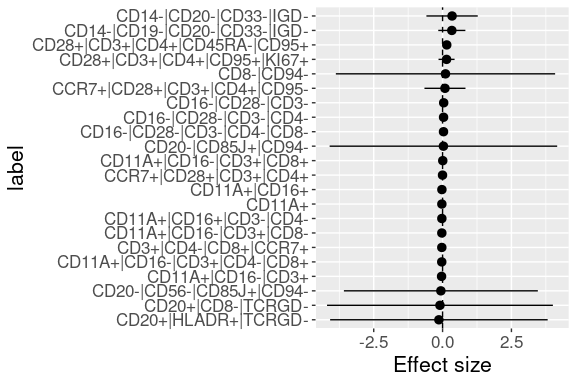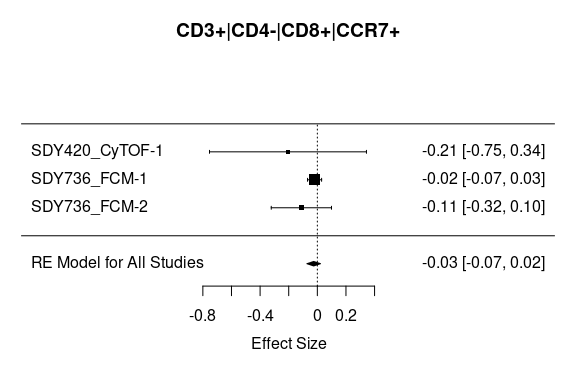# Introduction to MetaCyto

#### 2023-04-25

MetaCyto is an R package that performs meta-analysis of both flow cytometry and mass cytometry (CyTOF) data. It is able to jointly analyze cytometry data from different studies with diverse sets of markers. MetaCyto carries out the meta-analysis in 4 steps: data collection, data pre-processing, identifying common cell subsets across studies and statistical analysis. We created 2 examples to demonstrate the use of MetaCyto in this Vignette.In the first example, we provided an example to show how MetaCyto can be used to analyze your local cytometry datasets. The example will walk you through all 4 steps of meta-analysis. In the second example, we provide an example to show how to use functions in MetaCyto to automate the data collection step for datasets from ImmPort. More self-contained examples, including data and code, are available on GitHub (github.com/hzc363/MetaCyto_Examples).

• Example 1: Local Cytometry Datasets. This example shows how to perform meta-analysis using MetaCyto on your local datasets.

• Example 2: ImmPort Cytometry Datasets. This example shows how to automate the data collection step for cytometry data downloaded from ImmPort. The rest of steps in performing meta-analysis on ImmPort data is exactly the same as Example 1.

## Example 1: Local Cytometry Datasets.

In this example, we perform meta-analysis on cytometry data from 2 studies. One study uses flow cytometry and another study uses CyTOF. We will go through the 4 steps: data collection, data pre-processing, identifying common cell subsets across studies and statistical analysis, to carry out the meta-analysis.

### Step 1: data collection

For your local datasets, the data collection has to be done manually (If you are analyzing data from ImmPort database, this step is automated, see example 2). You need to create two csv files. The first file (fcs_info.csv) contains the path (relative to working direction) of each fcs files and the study each fcs file belongs to. Here is an example:

fcs_files study_id
SDY420/ResultFiles/CyTOF_result/11059_cells_found.573523.fcs SDY420_CyTOF-1
SDY420/ResultFiles/CyTOF_result/11445_cells_found.573686.fcs SDY420_CyTOF-1
SDY420/ResultFiles/CyTOF_result/11462_cells_found.573690.fcs SDY420_CyTOF-1
SDY420/ResultFiles/CyTOF_result/11491-1_cells_found.573697.fcs SDY420_CyTOF-1
SDY736/ResultFiles/Flow_cytometry_result/Hs90208C20090205_00.583331.fcs SDY736_FCM-1
SDY736/ResultFiles/Flow_cytometry_result/Hs90210C20090205_00.583065.fcs SDY736_FCM-1

The second file (sample_info.csv) contains the information about the samples or subjects corresponding to each of the fcs files. In this example, we try to see how gender and age affect immune cells. So we will include gender and age in the file. The path of fcs files in fcs_info.csv and sample_info.csv must be the same.

fcs_files Subject Age Gender
SDY420/ResultFiles/CyTOF_result/11059_cells_found.573523.fcs 84.00 Male
SDY420/ResultFiles/CyTOF_result/11445_cells_found.573686.fcs 84.00 Male
SDY420/ResultFiles/CyTOF_result/11462_cells_found.573690.fcs 90.00 Male
SDY420/ResultFiles/CyTOF_result/11491-1_cells_found.573697.fcs 86.00 Female
SDY736/ResultFiles/Flow_cytometry_result/Hs90208C20090205_00.583331.fcs 79.72 Male
SDY736/ResultFiles/Flow_cytometry_result/Hs90210C20090205_00.583065.fcs 33.00 Female

### Step 2: data preprocessing

In this step, we perform transformation for both CyTOF and flow cytometry data using the “preprocessing.batch” function. The function also performs compensation for flow cytometry data.

Before running the “preprocessing.batch” function, we need to define the type of each fcs files (FCM or CyTOF). We also need to tell the function the transformation parameter b we wish to use for each fcs files. Arcsinh transformation is used in the “preprocessing.batch” function: f(x) = asinh (b*x). We recommend b = 1/8 for CyTOF data and b = 1/150 for flow cytometry data.

# allocate variables
b=assay=rep(NA,nrow(fcs_info))

# define transformation parameter for each fcs files
b[grepl("CyTOF",fcs_info$study_id)]=1/8 b[grepl("FCM",fcs_info$study_id)]=1/150

# define cytometry type for each fcs files
assay[grepl("CyTOF",fcs_info$study_id)]="CyTOF" assay[grepl("FCM",fcs_info$study_id)]="FCM"

As you can see, the type of fcs file is identified by the “study_id” column in the fcs_info.csv file. So, it is beneficial to name your study_id informatively, not just use “study 1”, “study 2”.

Now we can use the “preprocessing.batch” function to perform data pre-processing. The output is written to the “preprocess_output” folder.

library(MetaCyto)

# find example data in the MetaCyto package. You won't need this line when running your actual meta-analysis
fcs_info$fcs_files=system.file("extdata",fcs_info$fcs_files,package="MetaCyto")

# preprocessing the data
preprocessing.batch(inputMeta=fcs_info,
assay=assay,
b=b,
outpath="Example_Result/preprocess_output",
excludeTransformParameters=c("FSC-A","FSC-W","FSC-H","Time","Cell_length"))

After the preprocessing, let’s look at what markers are included in each cytometry panels. After running the “panelSummary” function, a table and a graph are generated (written in “Example_Result” folder).

# collect preprocessing information
files=list.files("Example_Result",pattern="processed_sample",recursive=TRUE,full.names=TRUE)
panel_info=collectData(files,longform=FALSE)

# analyze the panels
PS=panelSummary(panelInfo = panel_info,
folder = "Example_Result",
cluster=FALSE,
width=30,
height=20)
knitr::kable(head(PS))
SDY420_CyTOF-1 SDY736_FCM-1 SDY736_FCM-2
CCR7 1 1 1
CD11A 0 1 0
CD127 1 0 0
CD14 1 0 0
CD16 1 1 0
CD161 1 0 0

Let’s see if there are any inconsistency in the marker names. The names of the same marker in different panels need to be the same. otherwise, the result can’t be combined.

sort(rownames(PS))

 “CCR7” “CD11A” “CD127” “CD14” “CD16”
 “CD161” “CD19” “CD20” “CD24” “CD25”
 “CD27” “CD28” “CD3” “CD33” “CD38”
 “CD4” “CD45RA” “CD56” “CD57” “CD62L”
 “CD8” “CD85J” “CD8B” “CD94” “CD95”
 “CELL_LENGTH” “DEAD” “DNA1” “DNA2” “FSC-A”
 “HLADR” “IGD” “KI67” “LIVE” “SAMPLE_ID”
 “SSC-A” “TCRGD” “TIME”

We can see that CD8 is called both “CD8” and “CD8B” in cytometry panels. So let’s fix this problem using the “nameUpdator”" function.

nameUpdator(oldNames=c("CD8B"), newNames=c("CD8"), files=files)

### step 3: Identification of common clusters across studies

In MetaCyto, the clusters in cytometry data can be identified using two pipelines, clustering pipeline and searching pipeline. The two pipelines can be used separately or be used together. In this example, we use both pipelines to identify novel and known cell populations.

We first use clustering pipeline to identify cell subsets in an un-supervised way.

#define parameters that we don't want to cluster
excludeClusterParameters=c("FSC-A","FSC-W","FSC-H","SSC-A","SSC-W","SSC-H","Time",

# Find and label clusters in the data. The default cluster functions
# are FlowSOM.MC. Here, flowHC, a hiarachical clustering function is used instead
# to show how non-default functions can be used.
cluster_label=autoCluster.batch(preprocessOutputFolder="Example_Result/preprocess_output",
excludeClusterParameters=excludeClusterParameters,
labelQuantile=0.95,
clusterFunction=flowHC)

We then use the searching pipeline to identify a known cell population. To do that, we just need to add the cell definition of the cell population. For example, in addition to the cell populations identified by the “autoCluster.batch”, we want to see how age affects CCR7+ CD8 T cells.

cluster_label=c(cluster_label,"CD3+|CD4-|CD8+|CCR7+")

We then derive summary statistics (including the proportion and median fluorescence intensity) for all the cell subsets. The output is written to the “search_output” folder.

searchCluster.batch(preprocessOutputFolder="Example_Result/preprocess_output",
outpath="Example_Result/search_output",
clusterLabel=cluster_label)

We can visualize the auto-gating results using histograms. The gray histograms show the distribution of markers in all cells. The red histograms show the distribution of markers in the identified cell subset. The histograms for all identified cell subsets in all cytometry panels are saved as pdf in the “search_output” folder.### Step 4: Statistical analysis

In this example, we are interested in knowing what cell types are affected by age. Before running the statistical analysis, we need to put demographic information (age and gender) in the sample_info.csv file and the summary statistics of cell subsets together.

library(dplyr)
# Collect Summary statistics generated in step 3
files=list.files("Example_Result/search_output",pattern="cluster_stats_in_each_sample",recursive=TRUE,full.names=TRUE)
fcs_stats=collectData(files,longform=TRUE)

# Get sample information generated in step 1
fn=system.file("extdata","sample_info_vignette.csv",package="MetaCyto")

# find data in the MetaCyto package. You won't need this line when running your actual meta-analysis
sample_info$fcs_files=system.file("extdata",sample_info$fcs_files,package="MetaCyto")

# join the cluster summary statistics with sample information
all_data=inner_join(fcs_stats,sample_info,by="fcs_files")

To see how the fraction of cell subsets within the blood chance with age, we can use the glmAnalysis function. The following code performs a regression model: fraction ~ Subject Age + Gender + study_id. The “variableOfInterest” argument specifies the variable that a researcher is interested in. The function will only report the effect size for the “variableOfInterst”, which is Subject Age in this case. The “otherVariables” argument specifies other variables to be included in the regression. In this case, Gender is included to control for gender differences. the study ID variable will always be included to adjust for batch effects. the “parameter” variable specifies the type of summary statistics. In this case, we are interested in how age affects the fraction of cells. If a researcher is interested in how age affects CD28 expression, change “parameter” argument from “fraction” to “CD28”.

# See the fraction of what clusters are affected by age (while controlling for Gender)
GA=glmAnalysis(value="value",variableOfInterst="Subject Age",parameter="fraction",
otherVariables=c("Gender"),studyID="study_id",label="label",
data=all_data,CILevel=0.95,ifScale=c(TRUE,FALSE))
GA=GA[order(GA$Effect_size),] # To save space, only cell populations with short cell definitions are plotted GA$label=as.character(GA$label) w = which(nchar(GA$label)<30)
GA = GA[w,]

# plot the results
plotGA(GA)The metaAnalysis function allows us to see the detailed effect size estimated in each cytometry panels. Here, let’s look at how the proportion of “CD3+ CD4- CD8+ CCR7+” population change with age.

# Subset the data to only include effect size of age on the proportion of "CD3+ CD4- CD8+ CCR7+"
L="CD3+|CD4-|CD8+|CCR7+"
dat=subset(all_data,all_data$parameter_name=="fraction"& all_data$label==L)

# run the metaAnalysis function
MA=metaAnalysis(value="value",variableOfInterst="Subject Age",main=L,
otherVariables=c("Gender"),studyID="study_id",
data=dat,CILevel=0.95,ifScale=c(TRUE,FALSE))## Example 2: Data collection for ImmPort datasets

The cytometry datasets from ImmPort are associated with standardized meta-data. Therefore, the fcs_info.csv and sample_info.csv can be generated automatically.

We first generate fcs info using the “fcsInfoParser” function for SDY736 from ImmPort.

# read meta-data of SDY736
fn=system.file("extdata","SDY736/SDY736-DR19_Subject_2_Flow_cytometry_result.txt",package="MetaCyto")

# Organize fcs file into panels
fn=system.file("extdata","SDY736",package="MetaCyto")
fcsCol="File Name",assay="FCM")

We then generate fcs info for SDY420

# read meta-data of SDY420
fn=system.file("extdata","SDY420/SDY420-DR19_Subject_2_CyTOF_result.txt",package="MetaCyto")

# Organize fcs file into panels
fn=system.file("extdata","SDY420",package="MetaCyto")
fcsCol="File Name",assay="CyTOF")

Then combine the fcs info for 2 studies

# Combine fcs info
fcs_info=rbind(fcs_info_SDY420,fcs_info_SDY736)

For generating sample info, we can use the “sampleInfoParser” function. We first generate sample info using for SDY736 from ImmPort.

# read meta-data of SDY736
fn=system.file("extdata","SDY736/SDY736-DR19_Subject_2_Flow_cytometry_result.txt",package="MetaCyto")

# Find the AGE, Gender info from selected_data
fn=system.file("extdata","SDY736",package="MetaCyto")
studyFolder=fn,
assay="FCM",
fcsCol="File Name",
attrCol=c("Subject Age","Gender"))

We then generate sample info for SDY420

# read meta-data of SDY420
fn=system.file("extdata","SDY420/SDY420-DR19_Subject_2_CyTOF_result.txt",package="MetaCyto")

# Find the AGE, Gender info from selected_data
fn=system.file("extdata","SDY736",package="MetaCyto")
attrCol=c("Subject Age","Gender"))
# Combine sample info
sample_info=rbind(sample_info_SDY420,sample_info_SDY736)